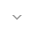# Keyframe

`public abstract class Keyframe`
`extends Object` `implements Cloneable`

 java.lang.Object ↳ android.animation.Keyframe

Keyframe类本身是抽象的。 特定于类型的工厂方法将返回特定于所存储值的类型的Keyframe的子类。 这是为了在处理最常见的情况下提高性能（例如， `float``int`值）。 其他类型将落入更一般的Keyframe类中，将其值视为Object。 除非动画需要处理需要直接动画的自定义类型或数据结构（并且使用`TypeEvaluator`的实现进行`TypeEvaluator` ），否则应该坚持使用float和int作为动画，使用这些类型的运行时间开销比其他类型低。

## Summary

### Public constructors

` Keyframe() `

### Public methods

` abstract Keyframe` ` clone() `

` float` ` getFraction() `

` TimeInterpolator` ` getInterpolator() `

` Class` ` getType() `

` abstract Object` ` getValue() `

` boolean` ` hasValue() `

` static Keyframe` ` ofFloat(float fraction) `

` static Keyframe` ` ofFloat(float fraction, float value) `

` static Keyframe` ` ofInt(float fraction, int value) `

` static Keyframe` ` ofInt(float fraction) `

` static Keyframe` ` ofObject(float fraction, Object value) `

` static Keyframe` ` ofObject(float fraction) `

` void` ` setFraction(float fraction) `

` void` ` setInterpolator(TimeInterpolator interpolator) `

` abstract void` ` setValue(Object value) `

### Inherited methodsFrom class ` java.lang.Object `

## Public constructors

### Keyframe

`Keyframe ()`

## Public methods

### clone

`Keyframe clone ()`

` x.clone() != x`
will be true, and that the expression:
` x.clone().getClass() == x.getClass()`
will be `true`, but these are not absolute requirements. While it is typically the case that:
` x.clone().equals(x)`
will be `true`, this is not an absolute requirement.

`Object`的方法`clone`执行特定的克隆操作。 首先，如果该对象的类没有实现接口`Cloneable` ，则引发`CloneNotSupportedException` 请注意，所有数组都被视为实现接口`Cloneable`并且数组类型`T[]``clone`方法的返回类型是`T[]` ，其中T是任何引用或基本类型。 否则，此方法创建该对象的类的新实例，并使用该对象的相应字段的内容来初始化其所有字段，就像通过赋值一样; 这些字段的内容本身并不克隆。 因此，此方法执行此对象的“浅拷贝”，而不是“深拷贝”操作。

`Object`本身并没有实现接口 `Cloneable` ，所以在类为 `Object`的对象上调用 `clone`方法将导致在运行时抛出异常。

Returns
`Keyframe` a clone of this instance.

### getFraction

`float getFraction ()`

Returns
`float` The time associated with this keyframe, as a fraction of the overall animation duration. This should be a value between 0 and 1.

### getInterpolator

`TimeInterpolator getInterpolator ()`

Returns
`TimeInterpolator` The optional interpolator for this Keyframe.

### getType

`Class getType ()`

Returns
`Class` The type of the value stored in the Keyframe.

### getValue

`Object getValue ()`

Returns
`Object` The value for this Keyframe.

### hasValue

`boolean hasValue ()`

Returns
`boolean` boolean Whether this object has a value assigned.

### ofFloat

`Keyframe ofFloat (float fraction)`

Parameters
`fraction` `float`: The time, expressed as a value between 0 and 1, representing the fraction of time elapsed of the overall animation duration.
Returns
`Keyframe`

### ofFloat

```Keyframe ofFloat (float fraction,
float value)```

Parameters
`fraction` `float`: The time, expressed as a value between 0 and 1, representing the fraction of time elapsed of the overall animation duration.
`value` `float`: The value that the object will animate to as the animation time approaches the time in this keyframe, and the the value animated from as the time passes the time in this keyframe.
Returns
`Keyframe`

### ofInt

```Keyframe ofInt (float fraction,
int value)```

Parameters
`fraction` `float`: The time, expressed as a value between 0 and 1, representing the fraction of time elapsed of the overall animation duration.
`value` `int`: The value that the object will animate to as the animation time approaches the time in this keyframe, and the the value animated from as the time passes the time in this keyframe.
Returns
`Keyframe`

### ofInt

`Keyframe ofInt (float fraction)`

Parameters
`fraction` `float`: The time, expressed as a value between 0 and 1, representing the fraction of time elapsed of the overall animation duration.
Returns
`Keyframe`

### ofObject

```Keyframe ofObject (float fraction,
Object value)```

Parameters
`fraction` `float`: The time, expressed as a value between 0 and 1, representing the fraction of time elapsed of the overall animation duration.
`value` `Object`: The value that the object will animate to as the animation time approaches the time in this keyframe, and the the value animated from as the time passes the time in this keyframe.
Returns
`Keyframe`

### ofObject

`Keyframe ofObject (float fraction)`

Parameters
`fraction` `float`: The time, expressed as a value between 0 and 1, representing the fraction of time elapsed of the overall animation duration.
Returns
`Keyframe`

### setFraction

`void setFraction (float fraction)`

Parameters
`fraction` `float`: time associated with this keyframe, as a fraction of the overall animation duration. This should be a value between 0 and 1.

### setInterpolator

`void setInterpolator (TimeInterpolator interpolator)`

Parameters
`interpolator` `TimeInterpolator`
Returns
`void` The optional interpolator for this Keyframe.

### setValue

`void setValue (Object value)`
`value` `Object`: value for this Keyframe.